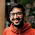## Friday, 13 July 2012

### Cadbury Pieces

A cadbury bar of m X n cm^2 has to be broken into pieces of 1X1 cm^2. Considering you can only break it either horizontally or vertically in one step. What is the minimum number of steps needed?

#### 9 comments:

1.m+n-4
first m-2 vertical breaks & then n-2 horizontal breaks

2.If you first do m-2 vertical breaks, then there would be m-1 pieces which then would be needed to be broken separately, so you can't break each of these pieces into smaller pieces in just n-2 horizontal breaks.

3.dynamic programming se solve kar sakte hai...

4.minSteps(x, y) = {
1 + minSteps(x/2, y)+minSteps(x-x/2, y) if(x > y)
1 + minSteps(x, y/2)+minSteps(x, y-y/2) else
}

with base case
minSteps(1, 1) = 0;

5.Well the solution is just too easy. Once a division is done, a new chocolate bar is formed and for that bar, you need to do an individual division. Ultimately you would end up with mXn pieces. This clearly implies you could not do any better than mXn-1 division. For understanding it in a better way, consider an example of 3X2 bar, no matter what strategy you apply, you need to make atleast 5 cuts in order to get 6 pieces.
Let us consider another question of same kind.

Consider 39 people participating a tournament. What would be the minimum number of matches required to find the winner?

6.min. no. of matches required 38... the argument is following: suppose M(n) denotes the min. no. of matches required to decide the winner for a group of n... now the winner for a larger group can be decided by adopting either of the two ways: (i) deciding winner for a smaller group k and then include that winner to the rest of the (n-k) and decide winner for (n-k+1)... the recursion is M(n)=M(k)+M(n-k+1)... and/or (ii) deciding winner for two smaller group k and (n-k) and finding winner between the two ... the recursion is M(n)=M(k)+M(n-k)+M(2) ... both the recursion have initial condition M(1)=0 & M(2)=1 ... the sol. is M(n) = n-1 ....

7.This comment has been removed by the author.

8.@rik : In both of your recursions, the fact that k can take values from 1 to n, is not reflected.

9.@sumit, k is smaller group than n, so it takes values from 1 to n... if you are talking about something else, then please elaborate....